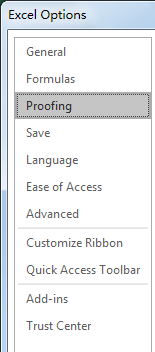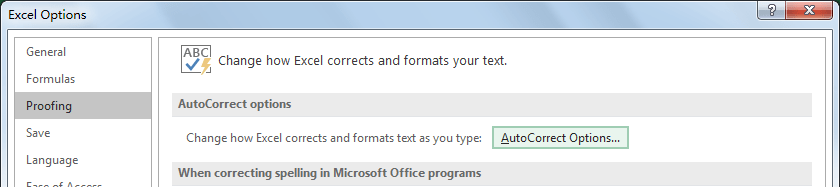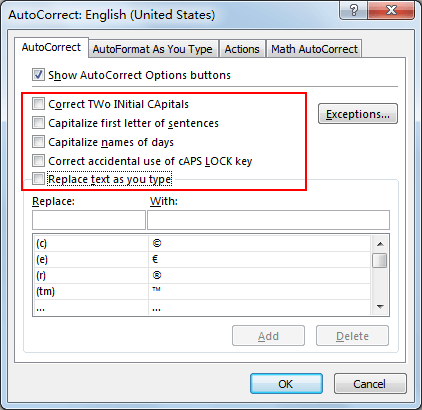# How to Turn Off AutoCorrect Feature in Excel?

In excel, when typing into the wrong spelled word, it will automatically correct it and display the correct word directly. This auto correct function is controlled by AutoCorrect feature. If we want to keep the wrong spelled word without changing, we can turn off the AutoCorrect feature. This tutorial will introduce you the way to turn off AutoCorrect, if you have this request you can follow below steps to turn off it.

## Method: Turn Off AutoCorrect Feature in Excel

When you typing ‘yuo’ in excel, it will be automatically corrected to ‘you’. This is because AutoCorrect feature is built in excel and it is enabled by default. Now let’s turn off it.

Step 1: Click File in excel ribbon, then click Options.Step 2: In ‘Excel Options’ window, click Proofing in the left panel.Step 3: Click AutoCorrect Options button under AutoCorrect options section.Step 4: In loaded AutoCorrect window, uncheck all AutoCorrect feature related options. Then click OK. In this step, you can also only check some options to disable part of AutoCorrect functions per your demand.

Step 5: Click OK to quit previous Excel Options window.

Step 6: Try to enter incorrect word like ‘yuo’ in any cell, you will find it will not be corrected to ‘you’ automatically. AutoCorrect feature is disabled now. If you want to enable it again, just repeat above steps to enter AutoCorrect window to enable all options.Related Posts

Calculate Number of Hours between Two Times

Calculating the difference between two times might be a valuable statistic for subsequent computations or averages, whether you're producing a time sheet for staff or recording personal exercises. While Excel has a plethora of complex functions, including date and time ...

Calculate Loan Interest in Given Year

When you borrow money, you are supposed to repay it gradually. Lenders, on the other hand, want to be compensated for their services and the risk they incur by lending you money. That is, you will not just repay the ...

Calculate Interest Rate for Loan

The interest rate is the fee charged by a lender to a borrower and is expressed as a percentage of the principal—the lent amount. The interest rate on a loan is often expressed as an annual percentage rate, abbreviated as ...

Calculate Interest for Given Period

Using the IPMT function in Excel, we can compute the interest payment on any loan. This step-by-step tutorial will guide Excel users of all skill levels through the process to calculate interest for given period. Finally, the formula: =IPMT(B3/12,1,B5,-B2) The ...

How To Use Excel GCD Function

This post will guide you how to use Excel GCD function with syntax and examples in Microsoft excel. Description The Excel GCD function Returns the greatest common divisor of two or more integers. So you can use the GCD function ...

Calculate A Ratio From Two Numbers In Excel

In elementary mathematics, a ratio is a connection or comparison between two or more integers. For example, ratios are often expressed as ":" to demonstrate the relationship between two numbers. You would think that manually calculating a ratio from two ...

How To Use Excel RRI Function

This post will guide you how to use Excel RRI function with syntax and examples in Microsoft excel. Description The Excel RRI function Returns an equivalent interest rate for the growth of an investment. So you can use the RRI ...

CAGR Formula Examples in Excel

CAGR in Excel is a formula that calculates the compound annual growth rate for any invested amount over the specified years or timeframe. Although there is no direct function in Excel that can help us identify the CAGR value, there ...

Build Hyperlink With VLOOKUP in Excel

You might have come across a task in which you were assigned to build hyperlinks, which seems very easy, and if you are new to excel or don't have enough experience with it, then you might wonder about doing this ...

Break ties with helper COUNTIF and column

Suppose you got a task to adjust the values that contain the ties; what would be your first attempt to break the ties of the given value? If you are wondering about doing this task manually, let me add that ...

Sidebar